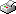Print This Page Jan Hagberg -- doctoral thesis -- On Degree Variance in Random Graphs Abstract This thesis is concerned with degree moments and degree variance in random graphs. The degree of vertex i in a graph is the number of edges incident to vertex i. In the first paper, degree moments and functions of degree moments are investigated for three random graph models. In statistical applications of random graph models the degree moments, and functions of the degree moments, have been found useful both as summary statistics and for inference on particular random graph models. Exact and asymptotic formulas are given for various degree statistics, in particular the degree variance. The second paper focus on the degree variance. Exact and asymptotic distributions of the degree variance are investigated for Bernoulli graphs and uniform random graphs. For graphs of large order, we show that the degree variance is approximately gamma distributed with parameters obtained from the first two moments of the degree variance. The usefulness of the results is illustrated by a graph centrality test with a critical value obtained from the gamma distribution. The third and last paper is concerned with extreme values and other attained values of the degree variance among graphs of fixed order and size, and among graphs of fixed order. The structure of the extreme graphs is investegated and it is shown that the maximum value of the degree variance can be obtained from integer sequences associated to the triangular numbers. Explicite formulas for the number of possible values and recurrence relations for the attained values of the degree variance are developed. Key words: Degree Sequences, Uniform Random Graphs, Bernoulli Graphs, Degree Moments, Degree Statistics, Degree Variance, Gamma Approximation, Centrality Testing, Integer Sequences Download report 1-->> General Moments of Degrees in Random Graphs Download report 2-->> Random Graph Distributions of Degree variance Download report 3-->> Extreme Values and Other Attained Values of the Degree Variance in Graphs